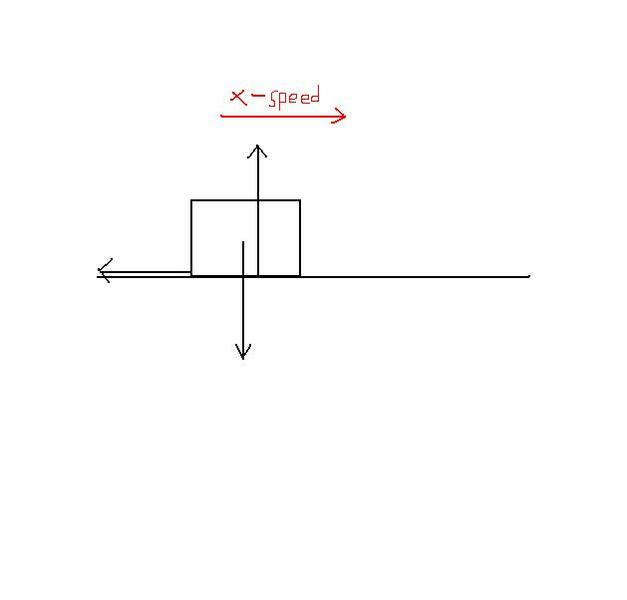# Weird physics question

## Homework Statement

A block, moving along a horizontal plank, is acted upon by a force of friction that is equal to 35% of the weight of the block. The block comes to rest from a speed of x m/s. Find x.

v=u + at
F=F1 + F2

## The Attempt at a Solution

I can't even attempt this because I have no clue where to start. They give you basically no information

Last edited:

## Homework Statement

A block, moving along a horizontal plank, is acted upon by a force that is equal to 35% of the weight of the block. The block comes to rest from a speed of x m/s. Find x.

v=u + at
F=F1 + F2

## The Attempt at a Solution

I can't even attempt this because I have no clue where to start. They give you basically no information

They give you information in the form of variables. They want an answer in the form of variables. Just to make sure, are they using x to represent speed, or x to represent distance traveled horizontally? This makes a difference.

They are using x to represent speed

Attempt (most likely wrong):

Equation to use: F=ma

You can find a relative mass (35% of the block weight) that stops the block. So that will =m. Force I don't know and a I don't know. I'm guessing you find a relative value of a which then can be used to find the speed.

They are using x to represent speed

Attempt (most likely wrong):

Equation to use: F=ma

You can find a relative mass (35% of the block weight) that stops the block. So that will =m. Force I don't know and a I don't know. I'm guessing you find a relative value of a which then can be used to find the speed.

Well you have two forces on the mass in the vertical, do you know what these are? They must be equal in magnitude and opposite in direction since the mass is not accelerating in the vertical.
And one force acting in the horizontal, friction. They are telling you that the frictional force is a portion of one of the forces in the vertical.

Here would be a picture of the forces assuming the mass is moving to the right. Do you know what the different forces are called. If so we can figure out a and get this done with some easy kinematics.The down force is the block pushing against the floor and the up force is the ground pushing back against the block (normal force), correct?

The down force is the block pushing against the floor and the up force is the ground pushing back against the block (normal force), correct?

Almost, the down force is due to gravity, mg... the earth pulling on the mass... and yes, the ground supplies the normal force back on the block. So now what can we say about the frictional force in terms of mg?

35%mg?

35%mg?

yep or 0.35mg which is equal to the frictioanl force which you already called ma. So now solve for a...

v=u + at

where v=final velocity=0, u=initial velocity, a=acceleration=.35mg, t=time=20 seconds

I forgot to add the time into the original question. My bad.

v=u + at

where v=final velocity=0, u=initial velocity, a=acceleration=.35mg, t=time=20 seconds

I forgot to add the time into the original question. My bad.

hold on...

0.35mg = ma so the m's cancel right? so after this you got a, v, t, and you are trying to determine u, which for some reason I cant figure is being called x? So you got everything you need.

So (.35)(9.8)=a, v=0, t=20 and then we get u :)

So (.35)(9.8)=a, v=0, t=20 and then we get u :)

yep...

so apparently friction is causing your mass to accelerate in the opposite direction it is moving bringing it to a halt v =0 over a 20 sec time period.

Yuppp I get it :d thank sooooo much